# Self-generating CONSORT diagram

library(consort)

The goal of this package is to make it easy to create CONSORT diagrams for the transparent reporting of participant allocation in randomized, controlled clinical trials. This is done by creating a standardized disposition data, and using this data as the source for the creation a standard CONSORT diagram. Human effort by supplying text labels on the node can also be achieved. Below is the illustration of the CONSORT diagram creating process for the two different methods.

## Prepare test data

In a clinical research, we normally will have a participants disposition data. One column is the participants’ ID, and the following columns indicating the status of the participants at different stage of the study. One can easily derive the number of participants at different stage by counting the number of participants on-study excluding the participants who are excluded.

set.seed(1001)
N <- 300

trialno <- sample(c(1000:2000), N)
exc1 <- rep(NA, N)
exc1[sample(1:N, 15)] <- sample(c("Sample not collected", "MRI not collected",
"Other"), 15, replace = T, prob = c(0.4, 0.4, 0.2))

induc <- rep(NA, N)
induc[is.na(exc1)] <- trialno[is.na(exc1)]

exc2 <- rep(NA, N)
exc2[sample(1:N, 20)] <- sample(c("Sample not collected", "Dead",
"Other"), 20, replace = T, prob = c(0.4, 0.4, 0.2))
exc2[is.na(induc)] <- NA

exc <- ifelse(is.na(exc2), exc1, exc2)

arm <- rep(NA, N)
arm[is.na(exc)] <- sample(c("Conc", "Seq"), sum(is.na(exc)), replace = T)
arm3 <- sample(c("Trt A", "Trt B", "Trt C"), N, replace = T)
arm3[is.na(arm)] <- NA

fow1 <- rep(NA, N)
fow1[!is.na(arm)] <- sample(c("Withdraw", "Discontinued", "Death", "Other", NA),
sum(!is.na(arm)), replace = T,
prob = c(0.05, 0.05, 0.05, 0.05, 0.8))
fow2 <- rep(NA, N)
fow2[!is.na(arm) & is.na(fow1)] <- sample(c("Protocol deviation", "Outcome missing", NA),
sum(!is.na(arm) & is.na(fow1)), replace = T,
prob = c(0.05, 0.05, 0.9))

df <- data.frame(trialno, exc1, induc, exc2, exc, arm, arm3, fow1, fow2)
rm(trialno, exc1, induc, exc2, exc, arm, arm3, fow1, fow2, N)

## Description

This function developed to populate consort diagram automatically. But to do so, a population disposition data should be prepared. The following data is prepared for demonstration.

• trailno: List of the subject ID.
• exc1: reason for exclusion after enrolled, should be missing if not excluded.
• induc: list of subject ID entered the induction phase.
• exc2: reason for exclusion in the induction phase, should be missing if not excluded.
• exc: reason for exclusion after enrolled. This is the combination of exc1 and exc2.
• arm: treatment arm, two groups.
• fow1: reason for exclusion in the follow-up, should be missing if not excluded.
• fow2: reason for exclusion in the final analysis, should be missing if not excluded.
• arm3: treatment arm, three groups.
#>   trialno exc1 induc exc2  exc  arm  arm3         fow1               fow2
#> 1    1086 <NA>  1086 <NA> <NA> Conc Trt C Discontinued               <NA>
#> 2    1418 <NA>  1418 <NA> <NA>  Seq Trt B         <NA>               <NA>
#> 3    1502 <NA>  1502 <NA> <NA>  Seq Trt C         <NA>               <NA>
#> 4    1846 <NA>  1846 <NA> <NA>  Seq Trt A         <NA>               <NA>
#> 5    1303 <NA>  1303 <NA> <NA>  Seq Trt A Discontinued               <NA>
#> 6    1838 <NA>  1838 <NA> <NA> Conc Trt B         <NA> Protocol deviation

# Usage

Basic logic:

1. The vertical node are the number of patients in the current node, no dropout reasons of inclusion reasons should be provided.
2. Side box is only used for the drop outs. It include information about the number of patients excluded and reasons.
3. Any subjects with exclusion reasons will not be included in the next vertical node box after excluded. So the subject id can be used multiple times to indicate how many patients left in the current node.
4. The node labels, for example visit number or phase, can only horizontally align to a vertical main nodes, not an exclusion box.
5. If more than 2 treatment allocation is present, all the exclusion box after the allocation will be aligned to the right

## Self-generating function

To generate consort diagram with data.frame, one should prepare a disposition data.frame.

consort_plot(data,
orders,
side_box,
allocation = NULL,
labels = NULL,
coords = NULL,
dist = 0.05,
cex = 0.8,
text_width = NULL,
widths = c(0.1, 0.9))
• data: Dataset prepared above
• orders: A named vector or a list, names as the variable and values as labels in the box. The order of the diagram will be based on this. Variables listed here, but not included in other parameters will calculate the number of non-missing values.
• side_box: Variable vector, appeared as side box in the diagram. The next box will be the subset of the missing values of these variables. The subject id variable can be used multiple times, since only the number of non-missing is calculated for the vertical box.
• allocation: Name of the grouping/treatment variable (optional), the diagram will split into branches on this variables.
• labels: Named vector, names is the location of the vertical node excluding the side box. The position location should plus 1 after the allocation variables if the allocation is defined.
• dist: Optional, distance between boxes. Default is 0.05.
• cex: Multiplier applied to font size, Default is 0.6
• coords: The horizontal coordinates for the nodes after split.
• text_width: A positive integer giving the target column for wrapping lines in the output.
• coords: A numeric vector of length 2 specifying relative percentage of the label and diagram in the final graph.

## Manual

Functions are mainly in three categories, main box, side box and label box. Others include building function. These are the functions used by the self generating function. These box functions require the previous node and text label. - add_box: add main box, no previous nodes should be provided if this is the first node. - add_side_box: add exclusion box. - add_split: add allocation box, all nodes will be split into groups. The label text for this node and following nodes should be a vector with a length larger than 1. - add_label_box: add visiting or phasing label given a reference node.

# Working example (self generation)

## Single arm

out <- consort_plot(data = df,
orders = c(trialno = "Population",
exc1    = "Excluded",
arm     = "Allocated",
fow1    = "Lost of Follow-up",
trialno = "Finished Followup",
fow2    = "Not evaluable for the final analysis",
trialno = "Final Analysis"),
side_box = c("exc1", "fow1", "fow2"),
cex = 0.9)
plot(out)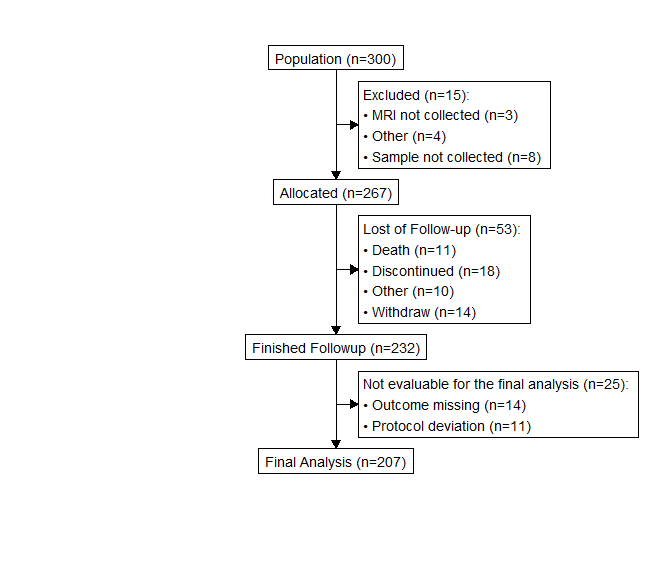## Two arms

out <- consort_plot(data = df,
orders = c(trialno = "Population",
exc    = "Excluded",
arm     = "Randomized patient",
fow1    = "Lost of Follow-up",
trialno = "Finished Followup",
fow2    = "Not evaluable",
trialno = "Final Analysis"),
side_box = c("exc", "fow1", "fow2"),
allocation = "arm",
coords = c(0.4, 0.6),
labels = c("1" = "Screening", "2" = "Randomization",
"5" = "Final"))

plot(out)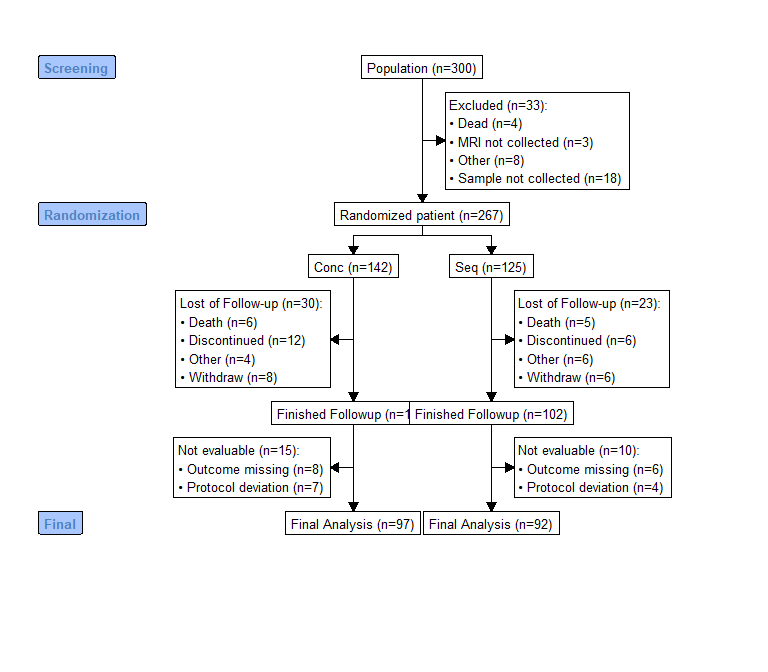## Three arms

g <- consort_plot(data = df,
orders = c(trialno = "Population",
exc    = "Excluded",
arm3     = "Randomized patient",
fow1    = "Lost of Follow-up",
trialno = "Finished Followup",
fow2    = "Not evaluable",
trialno = "Final Analysis"),
side_box = c("exc", "fow1", "fow2"),
allocation = "arm3",
labels = c("1" = "Screening", "2" = "Randomization",
"5" = "Final"))
plot(g)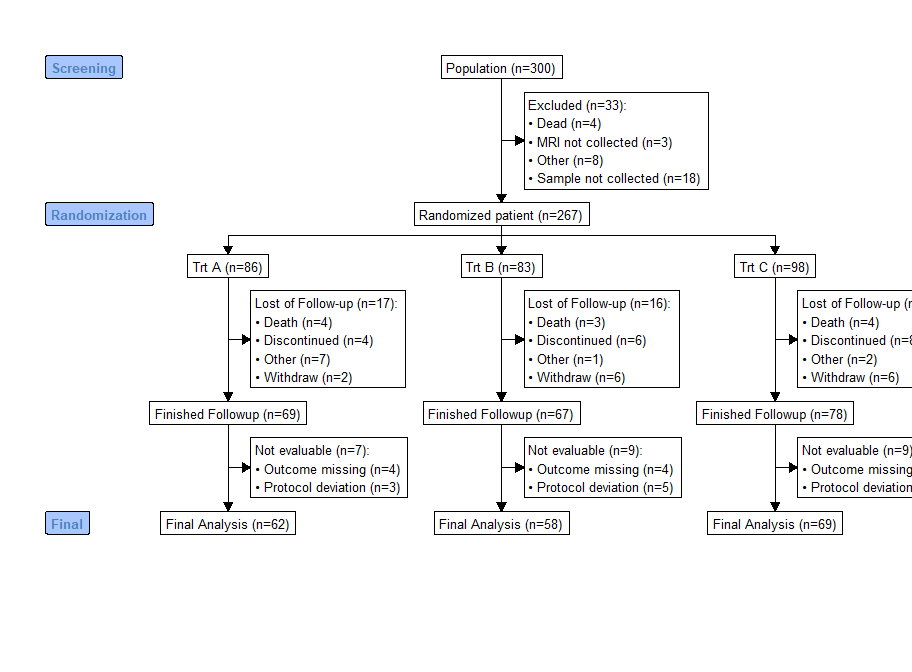## Multiple phase

g <- consort_plot(data = df,
orders = list(trialno = "Population",
exc1    = "Excluded",
induc   = "Induction",
exc2    = "Excluded",
arm3     = "Randomized patient",
fow1    = "Lost of Follow-up",
trialno = "Finished Followup",
fow2    = "Not evaluable",
trialno = "Final Analysis"),
side_box = c("exc1", "exc2", "fow1", "fow2"),
allocation = "arm3",
labels = c("1" = "Screening", "2" = "Month 4",
"3" = "Randomization", "5" = "Month 24",
"6" = "End of study"),
dist = 0.02,
cex = 0.7)
plot(g)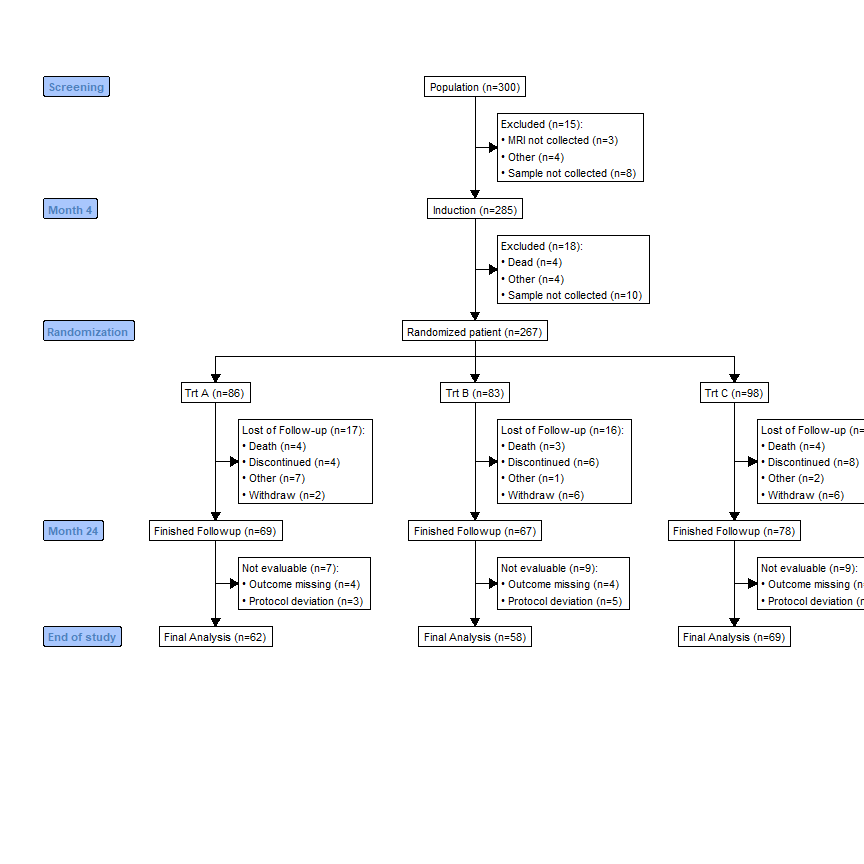# Working example (human effort)

The previous is to easily generate a consort diagram based on a disposition data, here we show how to create a consort diagram by providing the label text manually.

## Provide text

library(grid)
# Might want to change some settings
options(txt_gp = gpar(cex = 0.8))

txt1 <- "Population (n=300)"
txt1_side <- "Excluded (n=15):\n\u2022 MRI not collected (n=3)\n\u2022 Tissues not collected (n=4)\n\u2022 Other (n=8)"

# supports pipeline operator
g <- add_box(txt = txt1) |>
add_split(txt = c("Arm A (n=100)", "Arm B (n=100)")) |>
add_side_box(txt = c("Excluded (n=15):\n\u2022 MRI not collected (n=3)\n\u2022 Tissues not collected (n=4)\n\u2022 Other (n=8)",
"Excluded (n=7):\n\u2022 MRI not collected (n=3)\n\u2022 Tissues not collected (n=4)")) |>
add_box(txt = c("Final analysis (n=85)", "Final analysis (n=93)")) |>
"3" = "Randomized",
"4" = "Final analysis"))
plot(g)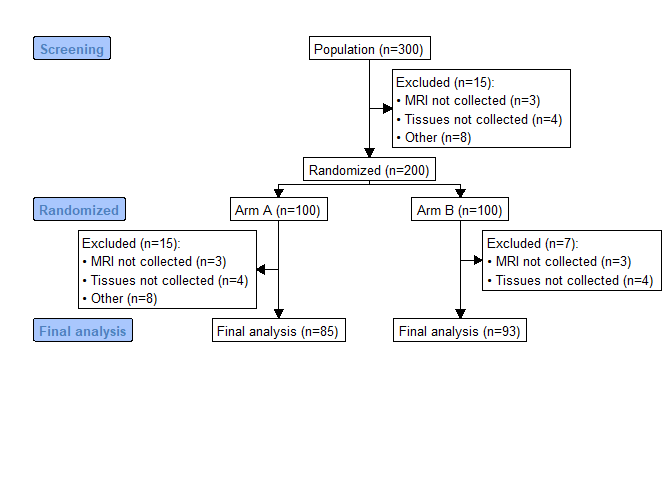## Using disposition table


df$arm <- factor(df$arm)

txt <- gen_text(df$trialno, label = "Patient consented") g <- add_box(txt = txt) txt <- gen_text(df$exc, label = "Excluded", bullet = TRUE)
g <- add_side_box(g, txt = txt)

# Exclude subjects
df <- df[is.na(df$exc), ] g <- add_box(g, txt = gen_text(df$arm, label = "Patients randomised"))

txt <- gen_text(df$arm) g <- add_split(g, txt = txt) txt <- gen_text(split(df$fow1, df$arm), label = "Lost to follow-up", bullet = TRUE) g <- add_box(g, txt = txt, just = "left") df <- df[is.na(df$fow1), ]
txt <- gen_text(split(df$trialno, df$arm),
label = "Primary analysis")
g <- add_box(g, txt = txt)

g <- add_label_box(g, txt = c("3" = "Baseline",
"4" = "First Stage"))

plot(g)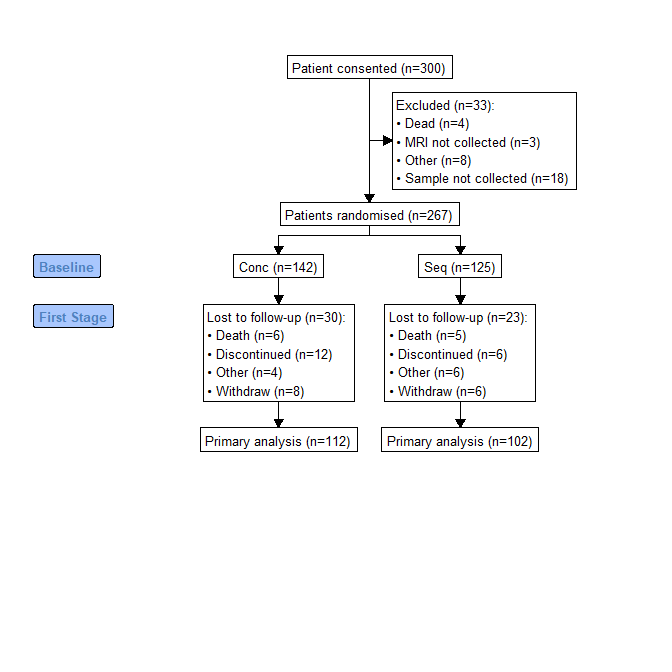# Saving plot

In order to export the plot to fit a page properly, you need to provide the width and height of the output plot. You might need to try different width and height to get a satisfying plot.You can use R basic device destination for the output. Below is how to save a plot in png format:

# save plots
png("consort_diagram.png", width = 29,
height = 21, res = 300, units = "cm", type = "cairo")
plot(g)
dev.off() 

Or you can use ggplot2::ggsave function to save the plot object:

ggplot2::ggsave("consort_diagram.pdf", plot = g)